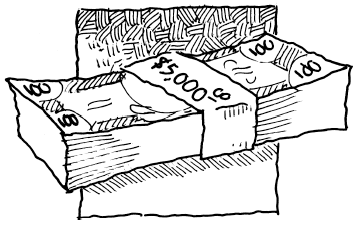### Home > PC3 > Chapter 5 > Lesson 5.1.1 > Problem5-5

5-5.Most investments receive compound interest as opposed to simple interest. This allows an investment to earn interest on the interest already received. Miranda plans to invest $\5000$. Calculate the value of her investment after one year, given the following rates

1. Annual interest of $12\%$ compounded monthly.

2. Annual interest of $8\%$ compounded quarterly ($4$ times per year).

3. Annual interest of $5.2\%$ compounded weekly.

4. Annual interest of $7.3\%$ compounded daily.

$A=P\left(1+\frac{r}{n}\right)^{nt}$

$A=$ final amount
$P=$ initial amount
$r=$ interest rate (as a decimal)
$n=$ number of compoundings per year
$t=$ time (years)